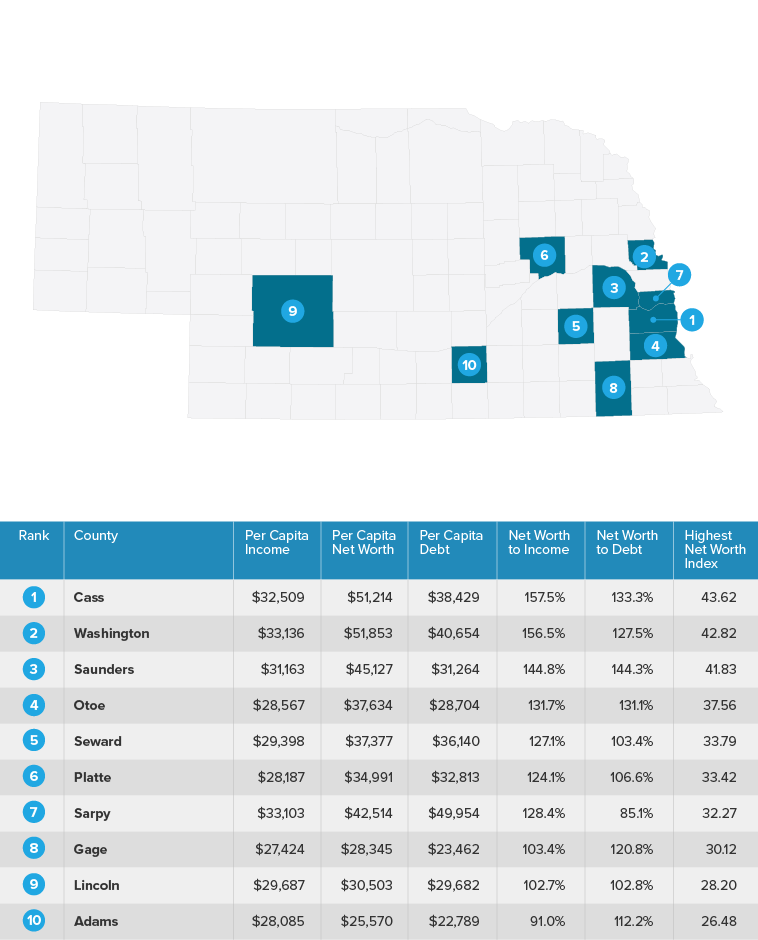# Places with the Highest Net Worth 2019 Nebraska

The below map highlights the places where people have the highest net worth. The study takes into account net worth as a percentage of income and net worth as a percentage of debt. Scroll below to view the data and methodology for how the study was researched and compiled.## Methodology

Our study aims to find the places in the United States where residents have the highest net worth. In order to do this we calculated two separate factors - net worth to income per capita and net worth to debt per capita.

First, we calculated the ratio of net worth to income per capita for every county. To achieve this, we divided the median household net worth by the average household size. We then divided that figure by the per capita income in each county. This number can serve as insight into how much people have saved or invested relative to their income.

We also calculated the ratio of net worth to debt per capita for each county. We calculated this figure by dividing the median household net worth by the average household size in each county. We then divided that amount by the per capita debt in each county.This measure incorporates a view of county residents’ debt burden relative to their net worth.

Finally, we created an overall index by combining the ratio of net worth to income per capita and net worth to debt per capita. The net worth to income index was given a weight of two and the net worth to debt index was given a weight of one. The counties with the highest overall index values represent those that have accumulated the highest net worth per capita, relative to income and debt, according to our study.

Sources: U.S. Census Bureau 2017 American Community Survey, ESRI, Federal Reserve Bank of New York